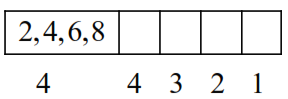# If the digits are not allowed to repeat in any number formed`
Question:

If the digits are not allowed to repeat in any number formed by using the digits $0,2,4,6,8$, then the number of all numbers greater than 10,000 is equal to

Solution:$=4 \times 4 \times 3 \times 2=96$